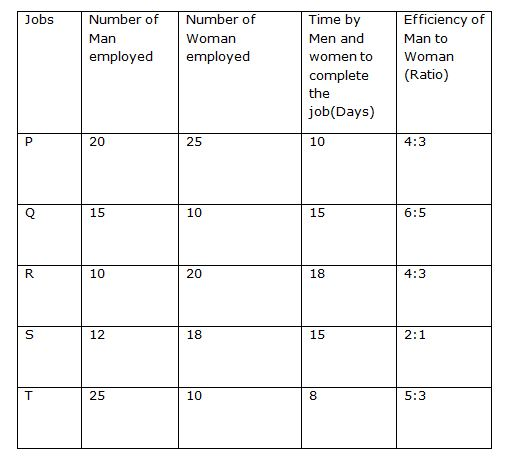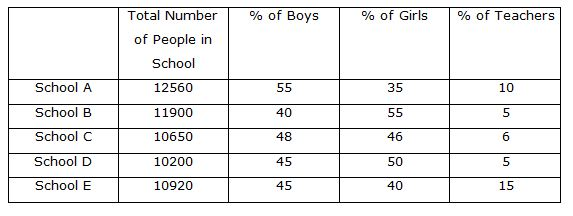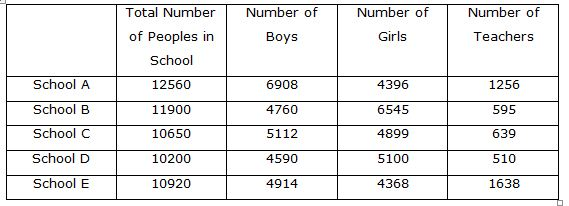# Quantitative Aptitude Questions (Data Interpretation) for SBI Clerk Mains 2018 Day-166

Dear Readers, SBI is conducting Online Examination for the recruitment of clerical cadre To enrich your preparation here we have providing new series of Data Interpretation – Quantitative Aptitude Questions. Candidates those who are appearing in SBI Clerk Mains Exams can practice these Quantitative Aptitude average questions daily and make your preparation effective.

[WpProQuiz 3078]

Click “Start Quiz” to attend these Questions and view Solutions

Directions (1-5): Study the following information carefully and answer the following question:1)  6 men and 9 women started the job P together but after 8 days 2 men left the work and 3 women joined, then in how many days the remaining job will be completed?

a) 24(3/70)

b) 21(23/26)

c) 10(1/19)

d) 21(25/26)

e) 48(3/8)

2) Job S was completed in 10 days with the help of 7 children then, find in how many days 6 women and 12children will complete job S?

a) 15

b) 30

c) 10

d) 40

e) 48

3) Due to urgency the job R need to be completed in 15 days so find how many more men should be employed to complete the work in 15 days?

a) 2

b) 3

c) 5

d) 4

e) 48

4) 15 men and 5 women started the job T together but after 10 days all the women left and the remaining work was completed by only men so find the wages received by each women for their contribution in the job if the total wages received by them is Rs 3720?

a) 24

b) 90

c) 10

d) 40

e) 48

5) If the efficiency ratio of man to children is 6:5. ‘X’ number of children’s were employed in job Q with the given men and women and the work got completed in 10 days so find the value of ‘X?

a) 14

b) 30

c) 10

d) 40

e) 48

Directions (6-10): Study the following table carefully and answer the questions given below it.6) Find the difference between the total number of girls in all the schools together and the total number of boys in all the schools together?

a) 956

b) 996

c) 985

d) 962

e) None of these

7) Number of girls from school A & total number of boys from School E together is approximately what percentage more/less than the total number of teachers in school E & total number of girls in school E together?

a) 55%

b) 68%

c) 72%

d) 43%

e) None of these

8) Find the difference between the girls from School C & School D together and Boys from School A &Teachers from school D?

a) 3571

b) 4289

c) 2609

d) 2581

e) None of these

9). Find difference between the average of total number of peoples in all the schools and the total number of teachers in all the schools together?

a) 820

b) 9560

c) 6608

d) 8246

e) None of these

10). The total number of girls from school C & total number of boys from School D together is approximately what percentage is the total number of teachers in school A & school B & school C ? (Approximately)

a) 199%

b) 256%

c) 323%

d) 153%

e) None of these

Total units of work in Job P= {(20*4) + (25*3)} * 10= 1550

Work done by 6 men and 9 women in 8 days= {(6*4) + (9*3)} * 8= 408

Remaining work= 1550- 408= 1142 units

Work done in one day by 4men and 12 women = {(4*4) + (12*3)}=52 units

So,

Number of days required= 1142/52= 21(25/26) days

Total units of work in job S= {(12*2) + (18*1)} *15= 630

Units of worked done in one day= 630/10= 63 units

Number of units of work done by 7 children

= 63- {(12*2) + (18*1)}

= 21 units

Number units done by one child= 21/7= 3 units

Units of work done by 6 women 12 children= {(6*1) + (12*3)} = 42

Number of days required= 630/42= 15 days.

Total units of work in job R= {(10*4) + (20*3)*18} = 1800

Number of units to be done in one day= 1800/15= 120 units

Let the number of extra men to be employed be x

(10+x)*4 + 20*3= 120

40+ 4x +60= 120

4x= 20

x= 5

No of extra men needed to complete the work in 15 days= 5 men

Total units of work in job T= {(25*5) + (10*3)*8} = 1240 units

Work done by 5 women in 10 days= (5*3)*10} = 150 units

Share of money received by 5 women = 150*3720/1240= Rs 450

Share of each women= 450/5= Rs 90

Total units of work in job Q= {(15*6) + (10*5)*15} = 2100

Number of unit of work done in one day= 2100/10= 210 units

So,

= {(15*6) + (10*5) + (x*5) = 210

= 90+50+ 5x= 210

= 5x= 70

= x= 14

So, number of children employed = 14

Directions (6-10):Number of girls = 4396 + 6545 + 4899 + 5100 + 4368 = 25308

Number of boys = 6908 + 4760 + 5112 + 4590 + 4914 = 26284

Required difference = 25308 – 26284 = 976

Total number of girls from school A & total number of boys from School E together = 4396 + 4914 = 9310

Total number of teachers in school E & total number of girls in school E together = 1638 + 4368 = 6006

Required % = [(9310 – 6006) / 6006] * 100 = 55% more

Number of girls from School C & School D = 4899 + 5100 = 9999

Number of Boys from School A = 6908

Number of Teachers from school D = 510

Required Difference = 9999 – 7418 = 2581

Average of total number of peoples in all the schools = (12560 +11900 + 10650 + 10200 + 10920) / 5 = 11246

Total number of teachers in all the Schools together

= 1256 + 595 + 639 + 510 + 1638 = 4638

Required Difference = 11246 – 4638 = 6608

Required total = 4899 + 4590 = 9489

Total number of teachers in school A & school B & school C = 1256 + 595 + 639 = 2490

Required % = (9489 / 2490) * 100 = 381%

Daily Practice Test Schedule | Good Luck

 Topic Daily Publishing Time Daily News Papers & Editorials 8.00 AM Current Affairs Quiz 9.00 AM Quantitative Aptitude “20-20” 11.00 AM Vocabulary (Based on The Hindu) 12.00 PM General Awareness “20-20” 1.00 PM English Language “20-20” 2.00 PM Reasoning Puzzles & Seating 4.00 PM Daily Current Affairs Updates 5.00 PM Data Interpretation / Application Sums (Topic Wise) 6.00 PM Reasoning Ability “20-20” 7.00 PM English Language (New Pattern Questions) 8.00 PM